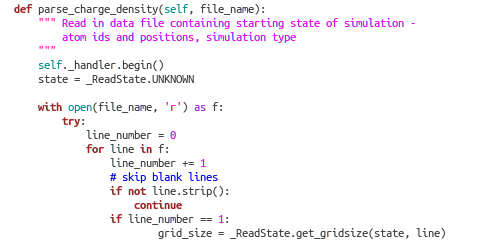QUANTUM ESPRESSO

QE is a simulator for calculation of electronic-structure properties using density functional theory (DFT), with a Plane-Wave (PW) basis set and pseudopotentials. The main routines pp.x and pw.x for pseudopotential and planewave calculations are supplemented by other packages for more specialized calculations including :

Energy barriers and reaction pathways (the 'NEB' method)

Vibrational properties

Codes and utilities for data postprocessing

Ballistic conductance

Spectra from time-dependent calculations

atomic calculations and pseudopotential generation.

Utilities for the calculation of projected density of states and free energy

Also available:

A gui for producing input data files for PWscf and some PostProc codes.

Phonon dispersion plotting

PLATFORMS

QE runs on Unix machines, including parallel machines using both OpenMP and MPI (Message Passing Interface) and GPU-accelerated machines. PC use possible thru 'cygwin' (unix under windows).

Requirements:

bash or tcsh, make, awk, sed. C and Fortran-95 compilers.

QE INPUT FILE FORMAT

example input file suitable for running a QE calculation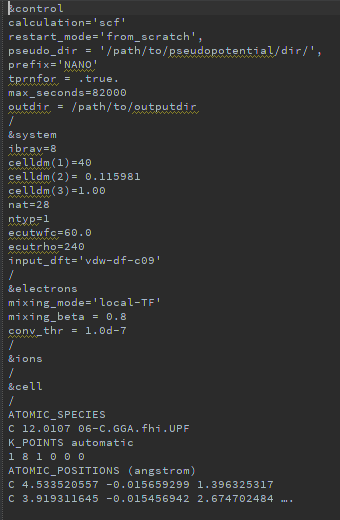&control – required parameters:

• Calculation - type of calculation, 'scf' is for self-consistent field.

• pseudo_dir - path to location for 'potential file' defining the potential field

• prefix - prefix for all files involved with a given calculation (pw.x and pp.x expect same prefix)

&system – required parameters:

• Ibrav – Bravais lattice index - 8 is orthorhombic, for instance. The basis vector depends on this; for orthorhombic the basis is x=(a,0,0), y=(0,b,0), z=(0,0,c)

• celldm : this part defines the crystallographic constants, in Bohr units (not in Angstrom; 1 Bohr = 0.529177249 Angstrom).

• nat : number of atoms in the simulation. It has to be equal to the number of lines under the "ATOMIC_POSITIONS" section.

• ntyp : defines the number of different types of atoms present in the simulation.

• ecutwfc : is the cutoff for the kinetic energy of the wavefunctions.

&electrons (optional): electronic variables, electron dynamics

&ions(optional): ionic variables, ionic dynamics

&cell (optional) : variable-cell dynamics

ATOMIC_SPECIES

Here you specify the type of atom, mass and name of the potential file used for this atom (located in 'pseudo_dir').

This brings us to

WRAPPER GOAL 1 – CONVERT QE INPUT FILE TO SIMPHONY DATA STRUCTURES

For this purpose, in simphony/simphony-quantum-espresso/simpony-espresso-dft/simespresso/io lives the file 'espresso_data_file_handler.py' which looks like: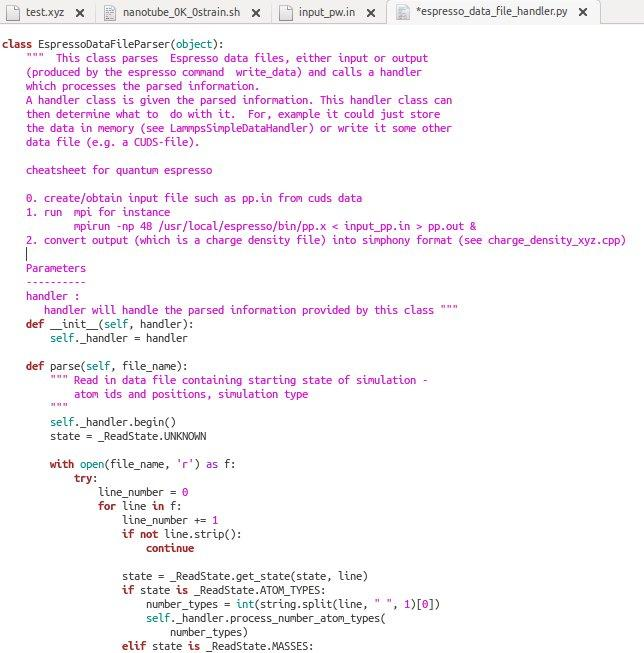&c. Currently only required fields are handled - many optional fields not handled.

WRAPPER GOAL 2 – CONVERT SIMPHONY DATA STRUCTURES TO QE INPUT FILES

Same as above but in opposite direction – takes simphony structures and outputs QE input file.

Once an input file is created, one can run one of the main QE routines pw.x using this input file. Using mpi this looks like :

mpirun -np n_processors /path/to/espresso/bin/pw.x < name.in > name.out

Wrappers can do this from python using 'subprocess':

subprocess.call(command_string,shell=True)

where command_string is the command you want. In this form , all the arguments can be tossed into the command string which obviates the need for tedious specification of the arguments otherwise.

Assuming this runs ok, the result is a folder with the name prefix.save, where prefix is the name indicatedin the input file, and an output file.

The 'bottom line' of these simulations is the electronic density. This is defined on a grid; to extract the charge density from the output file you create an input file for pp.x and get an output file with the charge density for each point of the grid.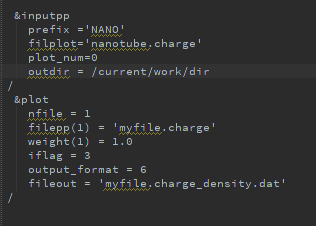&inputpp

• filplot : outputfile name

• plot_num : defines the quantity that one is interested in during the post-processing, 0 is for the charge density

&plot

• filepp(1) : name of the output file that will contain the quantity to be plotted and saved in fileout, here it is the charge density for example.

• output_format : an integer specifying the format of the output file .

• fileout : name of file containing the data to do the plot.

Run pp.x

Once this file is specified, one can run pp.x :

mpirun -n_processors x /usr/local/espresso/bin/pp.x< name.in > name.out

If all is ok, the output with the chage density is a file named name.charge. This file has the structure below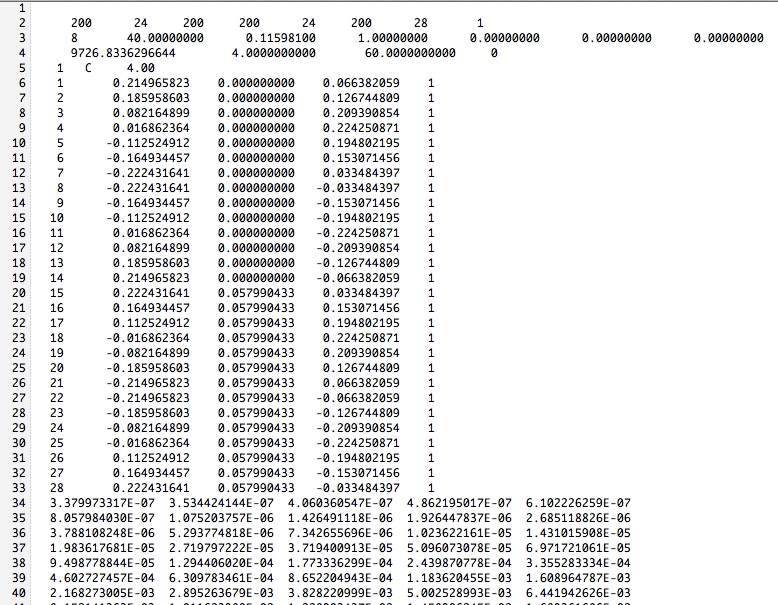First line

The first line has 8 numbers; the three first numbers are the size of the grid in the three spatial directions, and the three following are the same repeated. The seventh number corresponds to the number of atoms and the last one to the number of different type of atoms.
Second line

The first number is the type of Bravais lattice, and the three following numbers are the celldm defined in the input file of pw.x.

Next N_atom lines

These are atomic positions.

N_atom+1th line to end

This is what we are after, the charge density. Each value of charge density given in the output file corresponds to a point in the grid.

This takes us our third goal for QE wrappers:

WRAPPER GOAL 3 – CONVERT CHARGE DENSITY TO SIMPHONY 'ABCLATTICE'

Amongst others, the CUDS specifies a lattice data container which is suitable for holding the charge density from QE: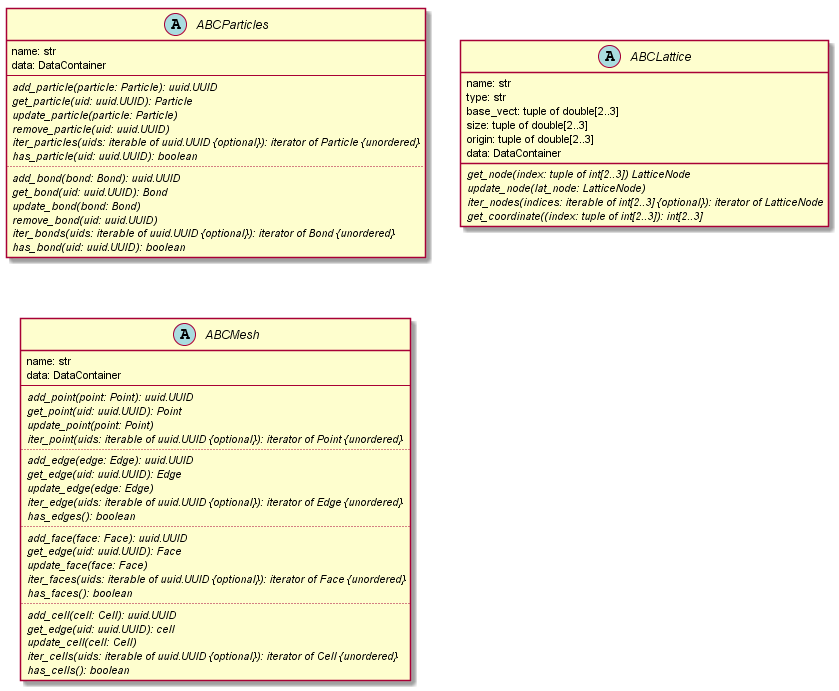The wrapper takes the charge density file as input and inserts the densitites into the CUDS data file.